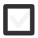# What is the ANGLE SUM of triangle & rectangle. What is the volume of the cone with 1m height & diameter.144 views
In 7-9
edited

## 2 lessons

+1 vote

Geometry

Practical excercices:

1. Go around your home and take pictures of things that represent the following (Google the names first if needed!):

• Cone
• Pyramid
• Rectangular prism
• Sphere
• Tetrahedron
• Cylinder
• Cuboid

2. Draw a floor plan of your dream house. Use at least the following shapes in your plan and name them in your drawing.

• Circle
• Square
• Rectangle
• Parallelogram
• Trapezoid

3. Practice using the compass by drawing these beautiful mandala shapes.

Let’s learn the theory:

Exercises:

1. Draw a circle and mark the following parts in it:

• Diameter
• Chord
• Circumference

2. Try to find as many objects around you that are shaped like a circle. Take some thread and measure their circumference and diameter. Plot the results in a graph (example below). What do you notice?

 Object Circum-ference Diameter Circum-ference/Diameter

3. Calculate the circumference of a circle when the radius is:

• 5,6 cm
• 12,6 m
• 1,0 km
• 0 m

4. The circumference of a circle is 74,2 m. Calculate:

• The diameter